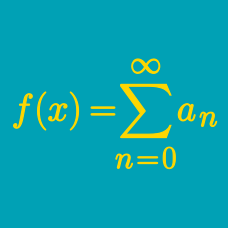Probability

# Generating Functions: Level 4 Challenges

Cody has 4 types of onions:

• The number of $\color{#69047E}\text{purple}$ onions can be any non-negative integer.
• The number of $\color{#20A900}\text{green}$ onions is a multiple of 2.
• The number of $\color{#D61F06}\text{red}$ onions is a multiple of 3.
• The number of $\color{#3D99F6}\text{blue}$ onions is a multiple of 5.

If Cody has 23 onions, how many different distributions of colors can there be?

A one year old bunny is sitting on the number 0 in the number line. His father, Bugs Bunny, is waiting for him on the number 10.

The bunny has to reach his father. At each minute, he can:

• Move one step forward;
• Move two steps forward;
• Stay still;
• Move one step backward;
• Move two steps backward.

There are $N$ possible ways the bunny can be on the number 10 after 10 minutes. Find the last three digits of $N$.

Details and assumptions

• The bunny is allowed to hop beyond 10, and then come back.

• There are no restrictions on the bunny entering the negative numbers. The bunny can go as much backwards as he wants.

• Order does matter. For example, the steps $\{0000022222\}$ and $\{2020202020\}$ are considered distinct.

How many polynomials $P(x)$ are there such that the coefficients of $P(x)$ are integers from 0 to 24 (inclusive) and $P(5) = 1200$?

Consider the recurrence relation $a_n = 2a_{n-1} + 3 a_{n-2} + 3^n$ for $n \geq 2$ with initial conditions $a_0 = -1, a_1 = 1.$

Given that $a_{100}$ is in the form of

$\LARGE \frac {x\cdot y^z - w}{v}$

where $x,y,w,z$ are prime numbers and $v$ as a perfect square, what is the value of $w+v+x+y+z$?

Find the general expression for $c_n$, where

$c_n - 3 c_{n-1} = 2 \cdot 5^n .$

×

Problem Loading...

Note Loading...

Set Loading...